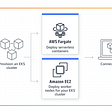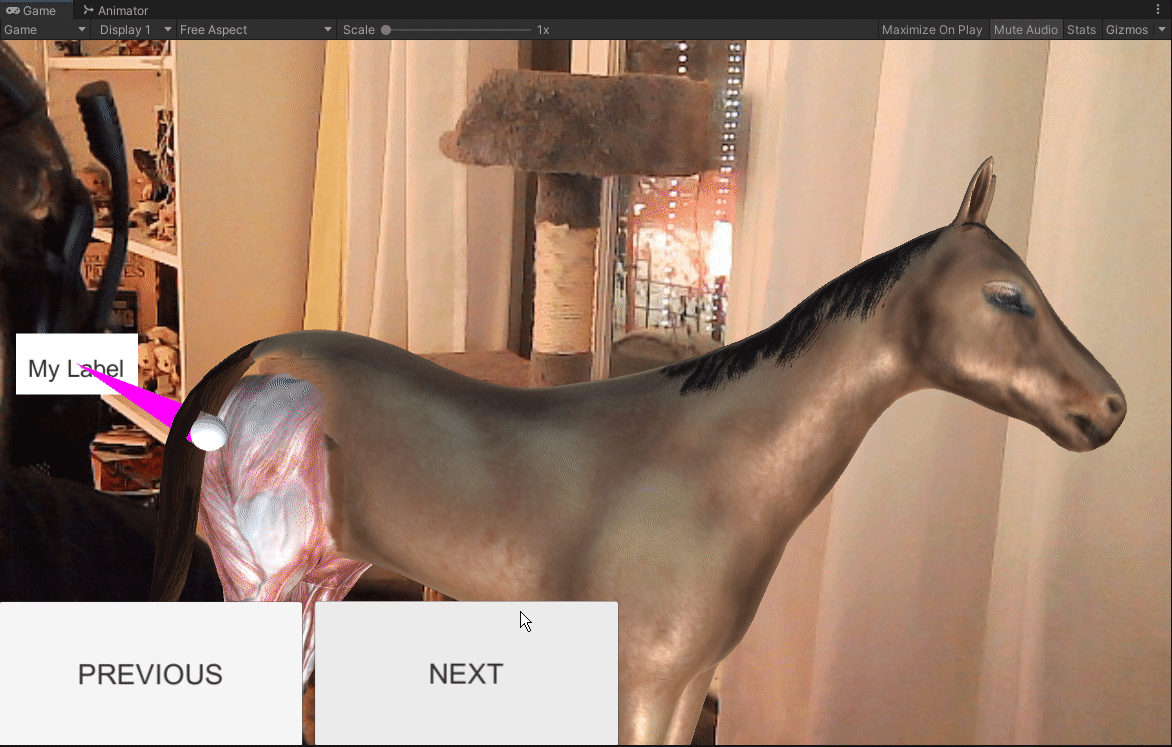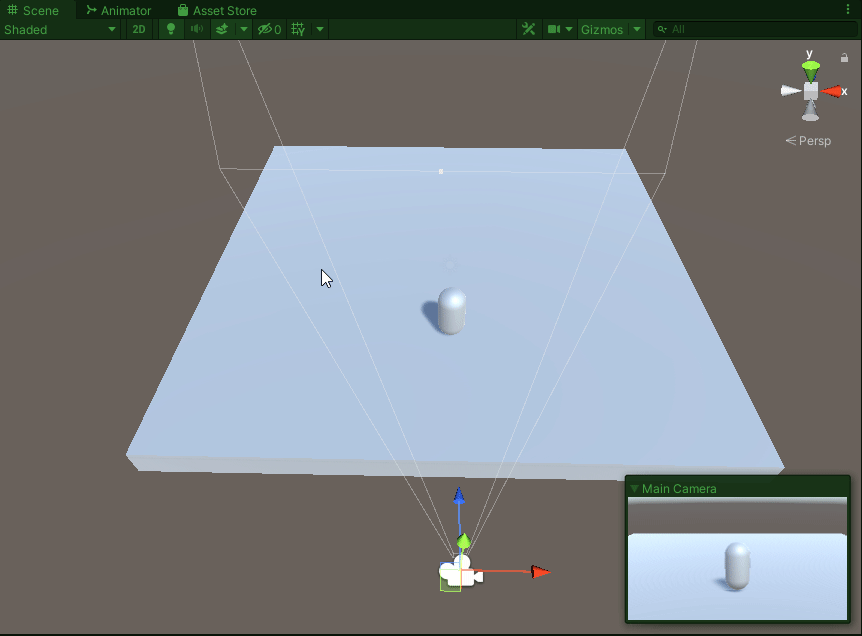# Understanding Big O

Now, let’s take a dive into Big O notation. Big O is the language that is used to talk about how long it takes to run an algorithm, or how much memory is used by an algorithm. Big O can express the best, worst and average case running time for an algorithm.
There are 4 main type of Big O notation that we will be looking at today:

1. 0(1)-Constant time → These will always take the same amount of time to be executed, and the amount of time is independent of the size of the input.
2. 0(n)-Linear time complexity → These algorithms time to execute are directly proportional to the input size. An example of which would be going through a stack of cards to find a certain one.
3. O(log n)-Logarithmic time complexity → This is an algorithm is an algorithm that is proportional to the input size of n. A quick example for this would be taking 8 cards from a deck. To find the 1 card you want of those 8, you would split it into 2 groups until you are left with just the 1 card.
4. O(n²)-Quadratic time complexity → For this algorithm, the number of steps to complete it is the value of n squared. For instance, if it normally 16 steps to complete a task, you would need to take 256 steps to complete it with this notation.

Now, let’s take a look at different examples that we can use to show how each differ from each other.

Let’s take a look at the first 2 scenarios. In this example, we want to look for the age 41 between the male and female groups. In the male group, it is a best case scenario, or O(1) because it happens to be the first age in the list. However, with our O(N) it isn’t a best case scenario as we have to scroll the the list a few times before we make it to 41:

As we can see, it took the count to reach 15 just to hit 41 in our list.

Now, let’s take a look at O(log n). As this is a binary search, we need to have our array sorted. What it will do from there is compare the element in the middle of the array with the target value and from there determines if the vale has been found or if it has to continue it’s search in either the top half or lower half of the array. With that in mind, let’s take a look how it would be with a longer array of numbers:

As we can see, we have built an array with 27 numbers in it that is sorted. If we decided to check it with our O(n) method, we would be looking at 18 different steps until we reach our target number. However, as we can see from this process, worked out by hand, in theory this process should only take 5 steps to achieve if we go through our O(log n) method. Let’s take a look at how we would go about writing this in code, and then check to see how many times our middle value pops up:

Now that we have our code written so we can compare our Linear to Binary search, let’s see the results:

As we can see, our Binary search took just 5 steps to complete as compared to the linear search of 18. This is roughly a 3rd of the amount of steps needed to reach our desired target.
Finally, let’s take a quick look at O(n²), or nested loops. What this can do is help us sort our lists so that we are able to create.
Let’s look at an example of how we can sort it by creating a random numbers sorted in numerical order:

What we have done is created a loop within our loop. This loop will then keep repeating itself until the list has been sorted. We need to create a temp value within the 2 loops so that when we need to switch the numbers, we have that temp value storing the switching numbers:

As we can see, upon pressing play, our elements within the array change from being completely random to becoming numerically sorted.

There we have it. This is a quick look into the process of the Big O notation. Next time we will take a quick look into another aspect, O(n log n) and see how this process stacks up to that of our O(log n).

--

--

--

## More from Nerd For Tech

NFT is an Educational Media House. Our mission is to bring the invaluable knowledge and experiences of experts from all over the world to the novice. To know more about us, visit https://www.nerdfortech.org/.

## Basic SQL queries in a nutshell## Web Application on AWS EKS:## New container capabilities in Red Hat Enterprise Linux 8.2## Develop Dynamic Flutter Applications by Following Web Standards## Kotlin Fundamentals in Examples## Azure DevOps Pipeline## Optimizing the Animator and Callouts## New Input System with Unity Learn — Lesson 1## Improving Upon Selection Highlighting in Our Game — Part 1## Local control for Player